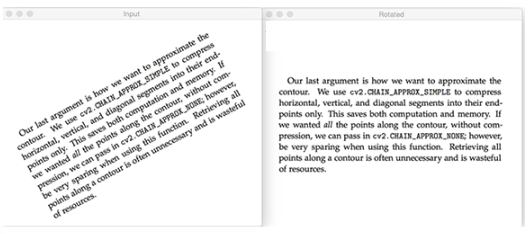# Detect and Correct the Text Skew Using Python OpenCV

In this tutorial, we will use an example to show you how to detect and correct the text skew using python opencv. you can learn how to do step by step.```import cv2
import numpy as np

2.Convert the image into a grayscale image

`gray_img=cv2.cvtColor(img, cv2.COLOR_BGR2GRAY)`

3.Invert the grayscale image

`gray_img=cv2.bitwise_not(gray_img)`

4.Select the x and y coordinates of the pixels greater than zero

`coordinates = np.column_stack(np.where(gray_img > 0))`

5.Calculate the skew angle

```ang=cv2.minAreaRect(coordinates)[-1]
if ang<-45:
ang=-(90+ang)
else:
ang=-ang```

6.Calculate the center of the text region

```height, width = img.shape[:2]
center_img = (width / 2, height / 2)```

7.Get the rotation matrix

```rotationMatrix = cv2.getRotationMatrix2D(center, angle, 1.0)
rotated_img = cv2.warpAffine(img, rotationMatrix, (width, height), borderMode = cv2.BORDER_REFLECT)```

8.Display the rotated image

```cv2.imshow("Rotated Image", rotated_img)
cv2.waitKey(0)```

Then, we will get the correct text skew.